# Community

Blog Events Webinars Tutorials Forum
×
Community Blog Image Search Explained – 2 More Methods

# Image Search Explained – 2 More Methods

In an earlier article titled "Principles of searching for similar images- Part 2", we introduced a method explaining how tools search for similar images.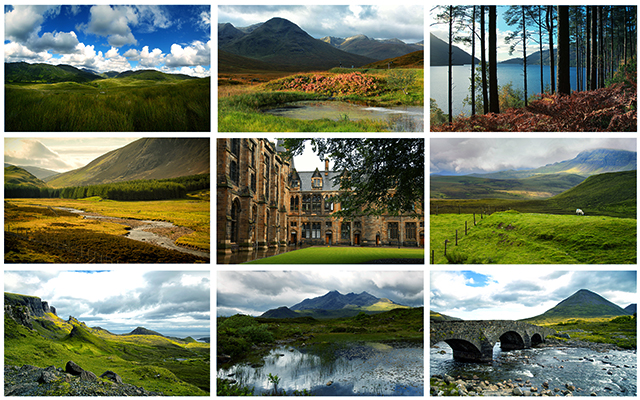In an earlier article titled "Image Search Explained – Method 1", we introduced a method explaining how tools search for similar images. In this post we discuss two more methods, the color distribution and the content feature method.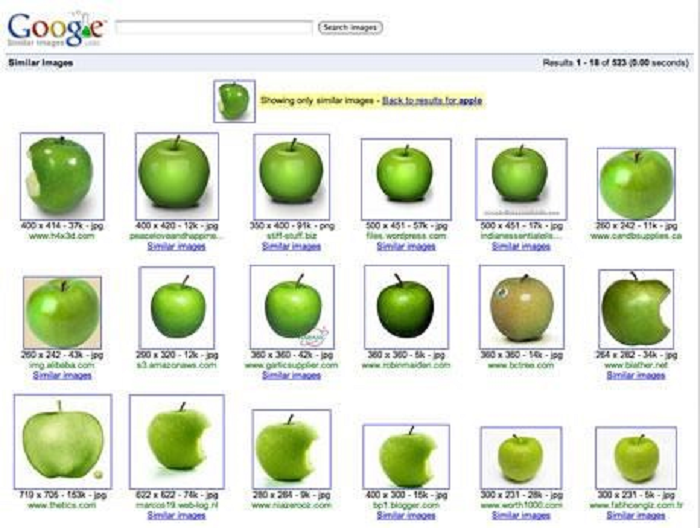## Color distribution method

You can generate a color histogram for each image. If the color histograms of two images are very close to each other, they are deemed similar.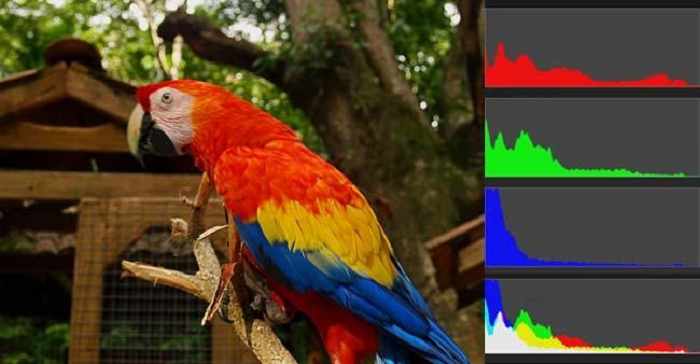Every color is made up of the three primary colors, red, green and blue (RGB). As such, four histograms exist (RGB histogram + the synthetic histogram).

If we successfully retrieve 256 values for every primary color, there will be 16 million different colors in the entire color space (256x256x256). It would involve a huge amount of computation to compare the histograms of these 16 million colors. We need a simplified method. We can divide the 0 - 255 range into four zones: 0 - 63 as Zone 0, 64 - 127 as Zone 1, 128 - 191 as Zone 2, and 192 - 255 as Zone 3. Ideally, this means that there are four zones for each of the red, green and blue color, so there are 64 combinations (4x4x4).

Any color must fall into one of the 64 combinations so that we can calculate the number of pixels contained in each combination.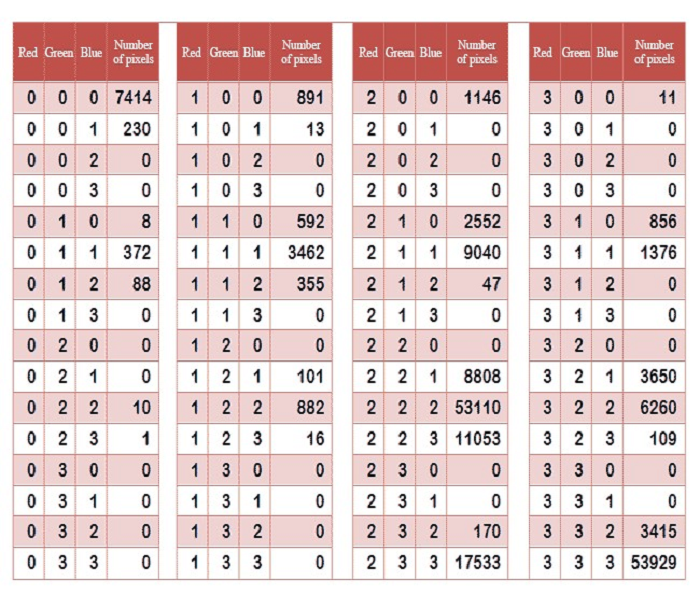The above table shows the color distribution of an image. Extract the bottom row of the table to form a 64-D vector (7414, 230, 0, 0, 8...109, 0, 0, 3415, 53929). This vector serves as the feature value of this image, also referred to as the image's "fingerprint."

Therefore, searching for similar images turns into finding out the similar vector. This is achievable by using the Pearson's correlation coefficient or cosine similarity.

## Content feature method

Apart from color composition, we can also compare the similarity of image content.

First, convert the original image into a smaller grayscale image, 50*50 pixels for example. Then determine a threshold value and convert the grayscale image into a black-and-white image.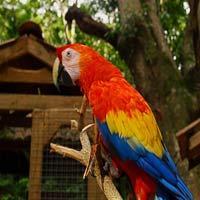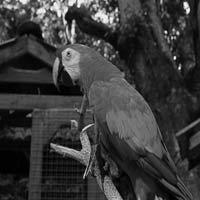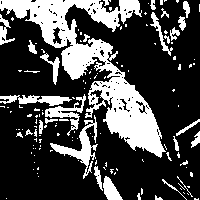If the two images are similar, their black-and-white outlines should be very close. As a result, the problem becomes how to identify a reasonable threshold value to present the outlines in the image correctly.

Apparently, the greater the contrast between the foreground and background colors, the clearer the outlines. This means we can find a value with which to minimize, or maximize, the intra-class variance of the foreground and background colors, and this value is the ideal threshold value.

In 1979, a Japanese scholar, Otsu, proved that the value minimizing the intra-class variance and that maximizing the inter-class variance refer to the same thing, namely the threshold value. He proposed a simple algorithm to evaluate this threshold value, known as the "Otsu's method". Here is how it works.

Suppose there are a total of n pixels in an image, among which n1 pixels have grayscale values smaller than the threshold value, and n2 pixels have grayscale values equal to or greater than the threshold value (n1 + n2 = n). W1 and W2 stand for the respective proportions of these two categories of pixels.

`w1 = n1 / n`

`w2 = n2 / n`

Now suppose the average value and variance of all pixels with the grayscale values smaller than the threshold value are μ1 and σ1 respectively, and those of all pixels with the grayscale values equal to or greater than the threshold value are μ2 and σ2 respectively. So we get:

`Intra-class variance = w1 (σ1 squared) + w2 (σ2 squared)`

`Inter-class variance = w1w2 (μ1-μ2)^2`

This proves that the two formulas are equivalent. The minimum value of "intra-class variance" is equal to the maximum value of "inter-class variance." However, the latter is easier to calculate.

Next, we will use the "method of exhaustion" to retrieve the threshold value from the minimum and maximum grayscale values and feed them into the formula above one by one. The specific example and Java algorithm is accessible here.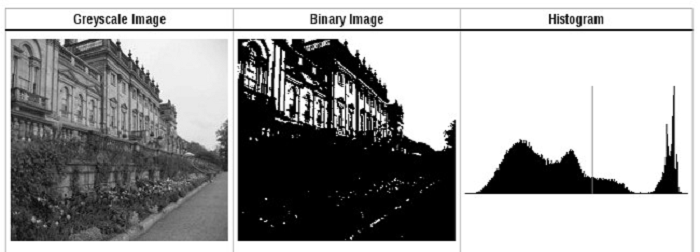The 50x50 pixels black-and-white thumbnail serves as a 50x50 0-1 matrix. Every value in the matrix corresponds to a pixel in the original image, with 0 representing black, and 1 representing white. This matrix is a feature matrix of the image.

Comparing the feature matrices of two images can help determine whether the images are similar. This can be achieved by using the "Exclusive - OR", or XOR operations. Following is the output table for this operation.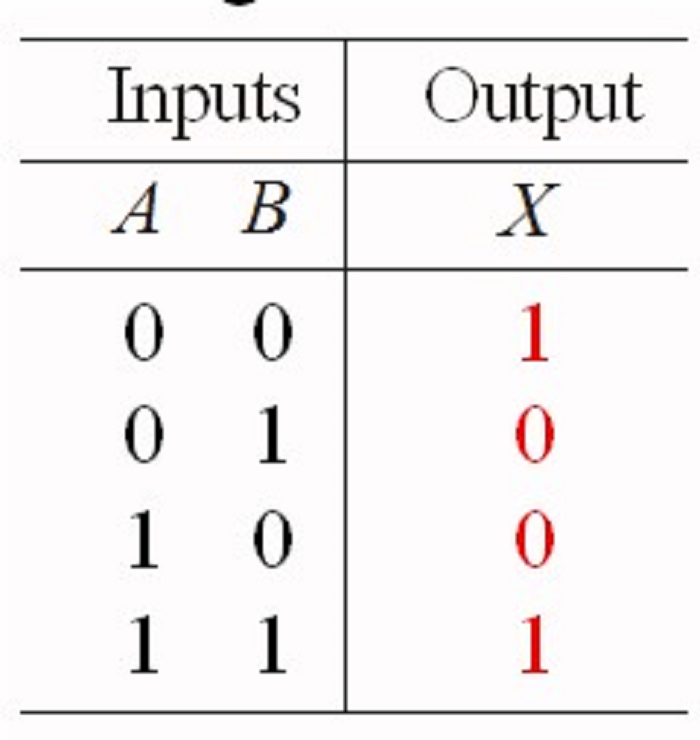As you can see, when the input values are same (either 0 or both 1), the output is 1, and when the input values are different, the output is 0.

Run the XOR operation for the feature matrices of different images. Imagine that the corresponding elements of the two feature matrices are the respective inputs A and B. Hence, more the "1s" in the result (indicating both matrices have 0 or 1), the more similar the images are.

1 1 1
Share on

### You may also like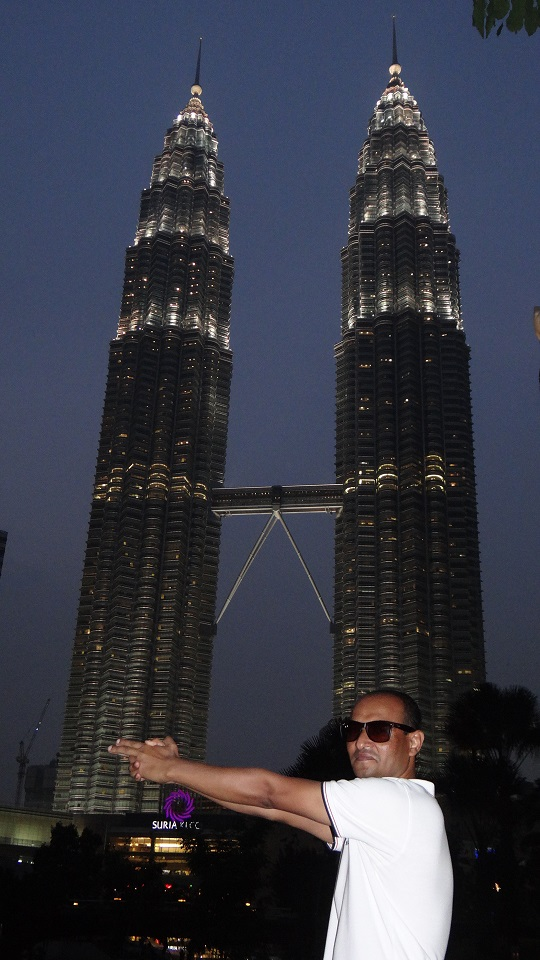## Raja_KT February 20, 2019 at 12:09 am

Segregating into ranges help to reduce the computing

# Related Products

• ##Image Search

An intelligent image search service with product search and generic search features to help users resolve image search requests.

• ##CT Image Analytics Solution

This technology can assist realizing quantitative analysis, speeding up CT image analytics, avoiding errors caused by fatigue and adjusting treatment plans in time.

• ##OpenSearch

OpenSearch helps develop intelligent search services.